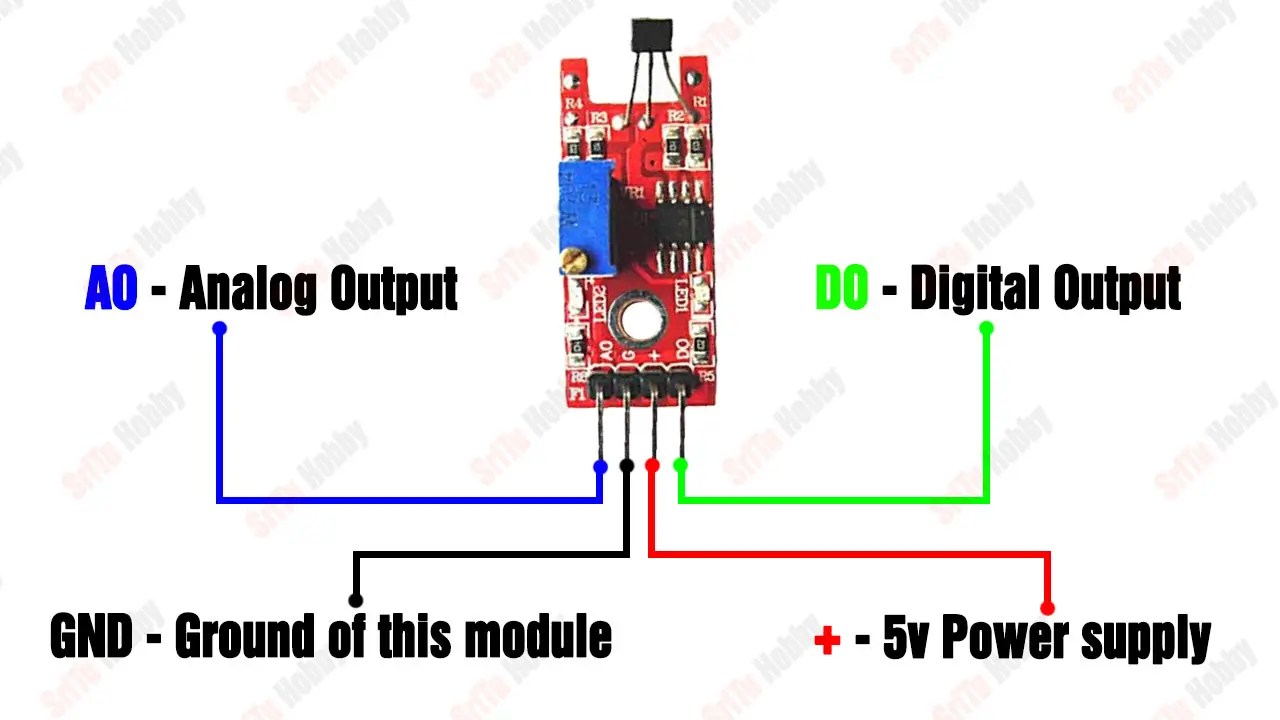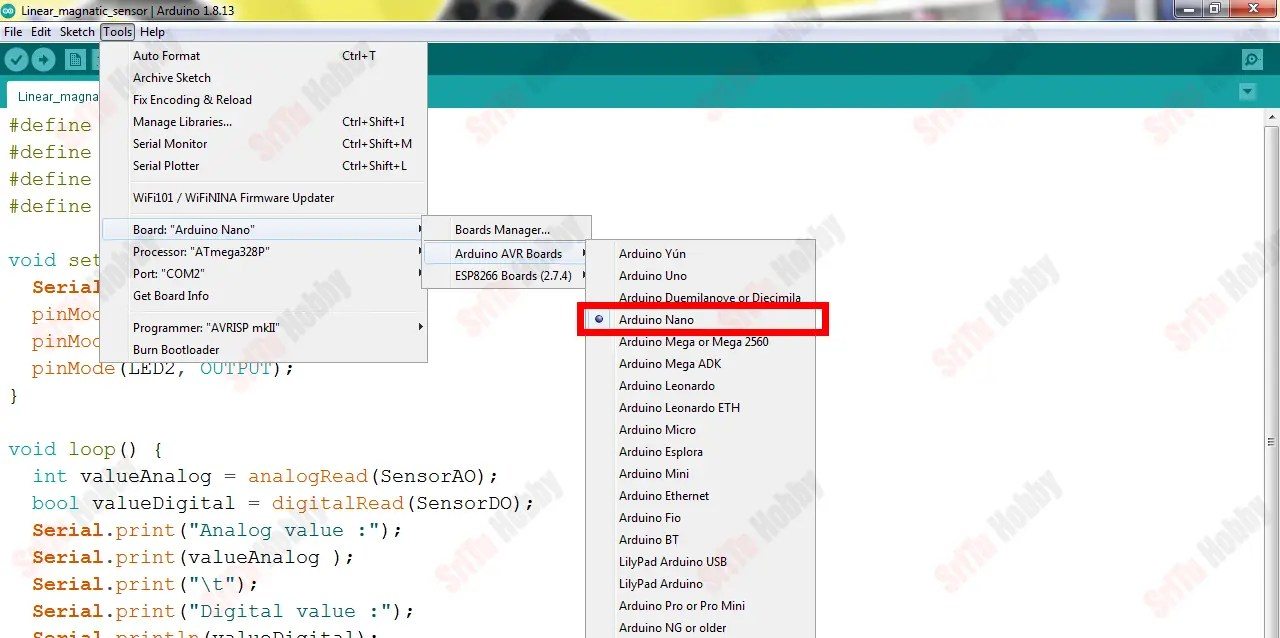# Linear hall effect sensor module with Arduino | How it works

Hello, welcome back. In this tutorial, we will learn what is the linear magnetic hall sensor and how to connect it to the Arduino. This sensor module is mainly designed using hall effect sensors. These are activated using a magnetic field. Also, these sensors are mainly used for distance measurement, anti-lock braking systems, proximity sensors, and switches. This hall effect sensor includes a rule called the hall effect.

### What is the hall effect?

When power is applied to a material, the current flow through it in a straight line. That is, the electrons travel in a straight line. Then, when a magnet is placed near that material, the electrons that traveled along with a straight line travel in a curved path again. This results in a potential difference or voltage from the permanent magnet and the electric current flow. This is mainly called the hall effect.

#### There are two types of hall effect sensors.

1.Digital output sensors.
2.Analog output sensors.

The ky-024 linear magnetic hall sensor module includes a hall effect sensor called 49E. Also, we can get digital and analog outputs using this sensor module. The potentiometer here can change the sensitivity of the output. The digital output can be used as a switch near a magnet, and the analog output can be used to measure the polarity and relative strength of a magnetic field.

##### PIN structure of this sensorOK, let’s learn how to connect this sensor with Arduino. The required components are as follows.

### Step 1

Firstly, identify these components.

### Step 2

Secondly, connect these components. To do this, use the circuit diagram below.### Step 3

Thirdly, let’s create the program for this project. It is as follows.

``````#define SensorAO A0
#define SensorDO 4
#define LED1 2
#define LED2 3

void setup() {
Serial.begin(9600);
pinMode(SensorDO, INPUT);
pinMode(LED1, OUTPUT);
pinMode(LED2, OUTPUT);
}

void loop() {
Serial.print("Analog value :");
Serial.print(valueAnalog );
Serial.print("\t");
Serial.print("Digital value :");
Serial.println(valueDigital);

if (valueDigital == 1) {
digitalWrite(LED1, HIGH);
digitalWrite(LED2, LOW);
} else if (valueDigital == 0) {
digitalWrite(LED2, HIGH);
digitalWrite(LED1, LOW);
} else {
digitalWrite(LED1, LOW);
digitalWrite(LED2, LOW);
}
}``````

#### Code explanation

Firstly, sensor pins and LED pins are defined.
#define SensorAO A0
#define SensorDO 4
#define LED1 2
#define LED2 3

In the setup function, these pins are set as output and input pins. Also, serial monitor is begin.
void setup() {
Serial.begin(9600);
pinMode(SensorDO, INPUT);
pinMode(LED1, OUTPUT);
pinMode(LED2, OUTPUT);
}

In the loop function, the analog and digital sensor values are read and entered into integers and Boolean variables.

After, these values are printed on the serial monitor.
Serial.print(“Analog value :”);
Serial.print(valueAnalog );
Serial.print(“\t”);
Serial.print(“Digital value :”);
Serial.println(valueDigital);

Next, the digital value is checked using the IF condition. If the value is 1, LED1 is activated. If the value is 0, LED2 is activated. Otherwise LED1 and LED2 will be inactive.
if (valueDigital == 1) {
digitalWrite(LED1, HIGH);
digitalWrite(LED2, LOW);
} else if (valueDigital == 0) {
digitalWrite(LED2, HIGH);
digitalWrite(LED1, LOW);
} else {
digitalWrite(LED1, LOW);
digitalWrite(LED2, LOW);
}

### Step 4

Lastly, select board and port. After, upload this code to the Arduino board.OK, enjoy this tutorial. The full video guide is given below. So, we will meet in the next tutorial.

Shopping Cart